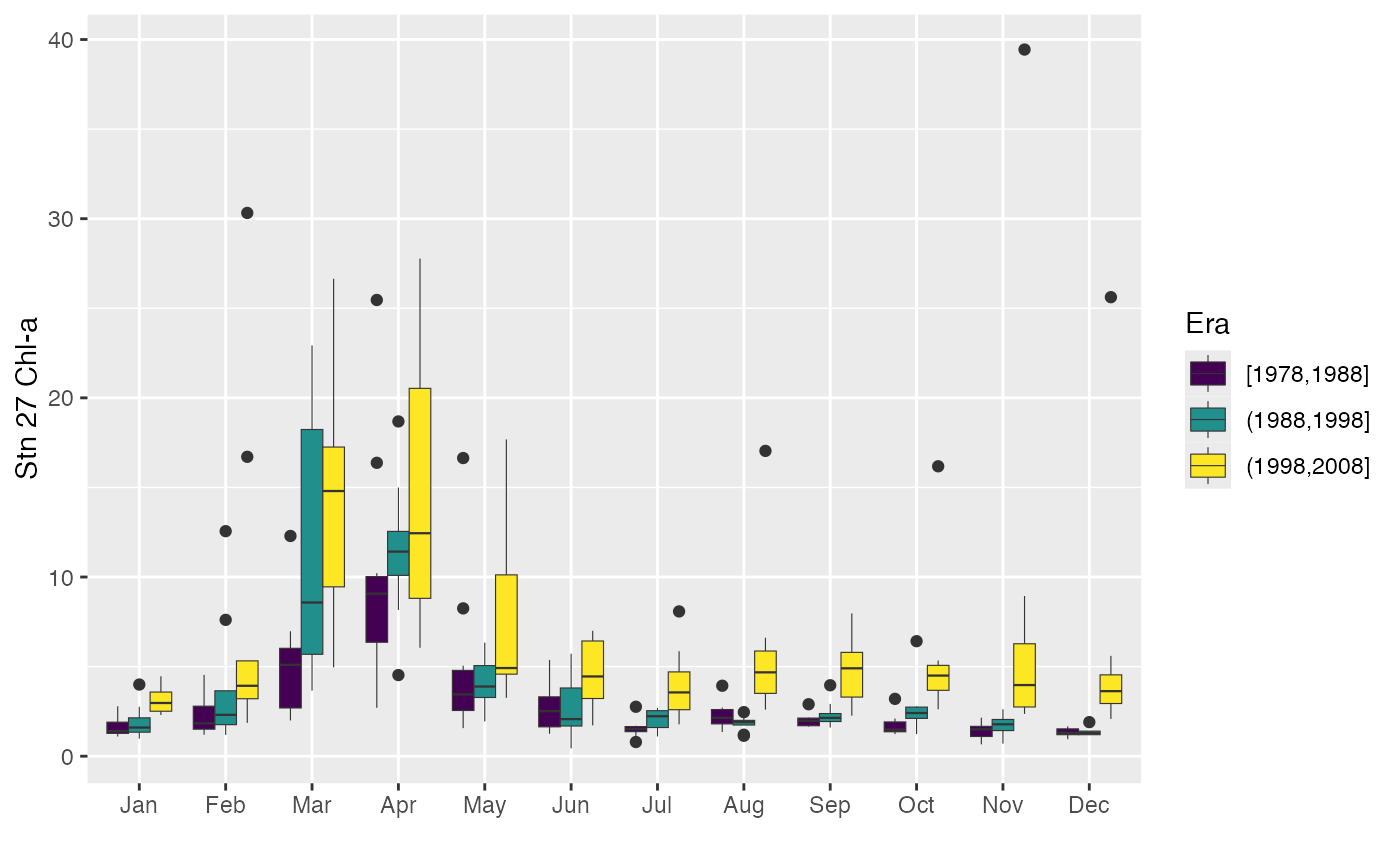Divides the time range for a monthly time series into different eras and plots composites of seasonal pattern. Can also plot each month separately for the entire record.

plotSeason(
x,
type = c("by.era", "by.month"),
num.era = 4,
same.plot = TRUE,
ylab = NULL,
num.col = 3
)

## Arguments

x Monthly time series Plot seasonal pattern by era, or each month for the entire record Integer number of eras, or vector of era year breaks Should eras be plotted by month? Optional character string label for y-axis Number of columns when plotted "by.month"

## Value

A plot (and the corresponding object of class "ggplot").

## Details

If num.era is an integer, the time range is divided into that many equal eras; otherwise, the time range is divided into eras determined by the num.era vector of years. When plotted "by.era" and same.plot = FALSE, the composite patterns are plotted in a horizontal row for easier comparison, which limits the number of periods that can be examined. Boxes based on fewer than half of the maximum possible years available are outlined in red. If same.plot = TRUE, a single plot is produced with era boxplots arranged by month. When plotted "by.month", values for each month are first converted to standardized anomalies, i.e., by subtraction of long-term mean and division by standard deviation. As always, and especially with these plots, experiment with the device aspect ratio and size to get the clearest information.

decompTs, seasonTrend

## Author

Alan Jassby, James Cloern

## Examples


chl27 <- sfbayChla[, 's27']
plotSeason(chl27, num.era = c(1978, 1988, 1998, 2008), ylab = 'Stn 27 Chl-a')plotSeason(chl27, num.era = 3, same.plot = FALSE, ylab = 'Stn 27 Chl-a')plotSeason(chl27, "by.month", ylab = 'Stn 27 Chl-a')